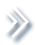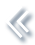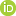####ArchivesReview Articles

## Cholesky-based model averaging for covariance matrix estimation

Hao Zheng ,

Gilead Sciences, Inc., Foster City, CA, USAKam-Wah Tsui ,Xiaoning Kang ,

International Business College, Dongbei University of Finance and Economics, Dalian, ChinaXinwei Deng

Department of Statistics, Virginia Tech, Blacksburg, VA, USAxdeng@vt.edu

Pages 48-58 | Received 01 Mar. 2017, Accepted 29 May. 2017, Published online: 28 Jul. 2017,
• Abstract
• References
• Citations

Estimation of large covariance matrices is of great importance in multivariate analysis. The modified Cholesky decomposition is a commonly used technique in covariance matrix estimation given a specific order of variables. However, information on the order of variables is often unknown, or cannot be reasonably assumed in practice. In this work, we propose a Cholesky-based model averaging approach of covariance matrix estimation for high dimensional data with proper regularisation imposed on the Cholesky factor matrix. The proposed method not only guarantees the positive definiteness of the covariance matrix estimate, but also is applicable in general situations without the order of variables being pre-specified. Numerical simulations are conducted to evaluate the performance of the proposed method in comparison with several other covariance matrix estimates. The advantage of our proposed method is further illustrated by a real case study of equity portfolio allocation.

• Bickel, P., & Levina, E. (2008a). Covariance regularization by thresholding. The Annals of Statistics, 36, 25772604[Google Scholar]
• Bickel, P., & Levina, E. (2008b). Regularized estimation of large covariance matrices. The Annals of Statistics, 36, 199227[Google Scholar]
• Bien, J., & Tibshirani, R. (2011). Sparse estimation of a covariance matrix. Biometrika, 98, 807820[Google Scholar]
• Burman, P. (1989). A comparative study of ordinary cross-validation, v-fold cross-validation and the repeated learning-testing methods. Biometrika, 76, 503514[Google Scholar]
• Chen, Z., & Leng, C. (2015). Local linear estimation of covariance matrices via Cholesky decomposition. Statistica Sinica, 25, 12491263[Google Scholar]
• Cochran, W. G. (1977). Sampling techniques. New York, NY: John Wiley & Sons.  [Google Scholar]
• Deng, X., & Tsui, K.-W. (2013). Penalized covariance matrix estimation using a Matrix-Logarithm transformation. Journal of Computational and Graphical Statistics, 22, 494512[Taylor & Francis Online]
• Fan, J., Xue, L., & Zou, H. (2016). Multitask quantile regression under the transnormal model. Journal of the American Statistical Association, 111, 17261735[Taylor & Francis Online]
• Friedman, J., Hastie, T., & Tibshirani, R. (2010). Regularization paths for generalized linear models via coordinate descent. Journal of Statistical Software, 33, 122[Google Scholar]
• Golub, G. H., & Van Loan, C. F. (2012). Matrix computations. Baltimore, MD: The Johns Hopkins University Press.  [Google Scholar]
• Hunter, D. R., & Lange, K. (2000). Quantile regression via an MM algorithm. Journal of Computational and Graphical Statistics, 9, 6077[Taylor & Francis Online]
• James, W., & Stein, C. (1961). Estimation with quadratic loss. Proceedings of the Fourth Berkeley Symposium on Mathematical Statistics and Probability, 1, 361379[Google Scholar]
• Johnstone, I. M. (2001). On the distribution of the largest eigenvalue in principal components analysis. The Annals of Statistics, 29, 295327[Google Scholar]
• Kullback, S., & Leibler, R. A. (1951). On information and sufficiency. The Annals of Mathematical Statistics, 22, 7986[Google Scholar]
• Ledoit, O., & Wolf, M. (2004). A well-conditioned estimator for large-dimensional covariance matrices. Journal of Multivariate Analysis, 88, 365411[Google Scholar]
• Levina, E., Rothman, A., & Zhu, J. (2008). Sparse estimation of large covariance matrices via a nested Lasso penalty. The Annals of Applied Statistics, 2, 245263[Google Scholar]
• Liu, H., Wang, L., & Zhao, T. (2013). Sparse covariance matrix estimation with eigenvalue constraints. Journal of Computational and Graphical Statistics, 23, 439459[Taylor & Francis Online]
• Markowitz, H. (1952). Portfolio selection. The Journal of Finance, 7, 7791[Google Scholar]
• Michaud, R. O. (1989). The Markowitz optimization enigma: Is ‘optimized’ optimal? Financial Analysts Journal, 45, 3142[Taylor & Francis Online]
• Pinheiro, J. C., & Bates, D. M. (1996). Unconstrained parametrizations for variance-covariance matrices. Statistics and Computing, 6, 289296[Google Scholar]
• Pourahmadi, M. (1999). Joint mean-covariance models with applications to longitudinal data: Unconstrained parameterisation. Biometrika, 86, 677690[Google Scholar]
• Rothman, A., Levina, E., & Zhu, J. (2010). A new approach to Cholesky-based covariance regularization in high dimensions. Biometrika, 97, 539550[Google Scholar]
• Trefethen, L. N., & Bau III, D. (1997). Numerical linear algebra. Philadelphia, PA: Society for Industrial and Applied Mathematics.  [Google Scholar]
• Wang, Y., & Daniels, M. (2014). Computationally efficient banding of large covariance matrices for ordered data and connections to banding the inverse Cholesky factor. Journal of multivariate analysis, 130, 2126[Google Scholar]
• Xue, L., Ma, S., & Zou, H. (2012). Positive definite l1 penalized estimation of large covariance matrices. Journal of the American Statistical Association, 107, 14801491[Taylor & Francis Online]
, . (2021) An improved banded estimation for large covariance matrix. Communications in Statistics - Theory and Methods 0:0, pages 1-17.
, . (2021) Intrinsic Wavelet Regression for Curves of Hermitian Positive Definite Matrices. Journal of the American Statistical Association 116:534, pages 819-832.
, , . (2020) A Cholesky-based estimation for large-dimensional covariance matrices. Journal of Applied Statistics 47:6, pages 1017-1030.
, . (2020) An improved modified cholesky decomposition approach for precision matrix estimation. Journal of Statistical Computation and Simulation 90:3, pages 443-464.